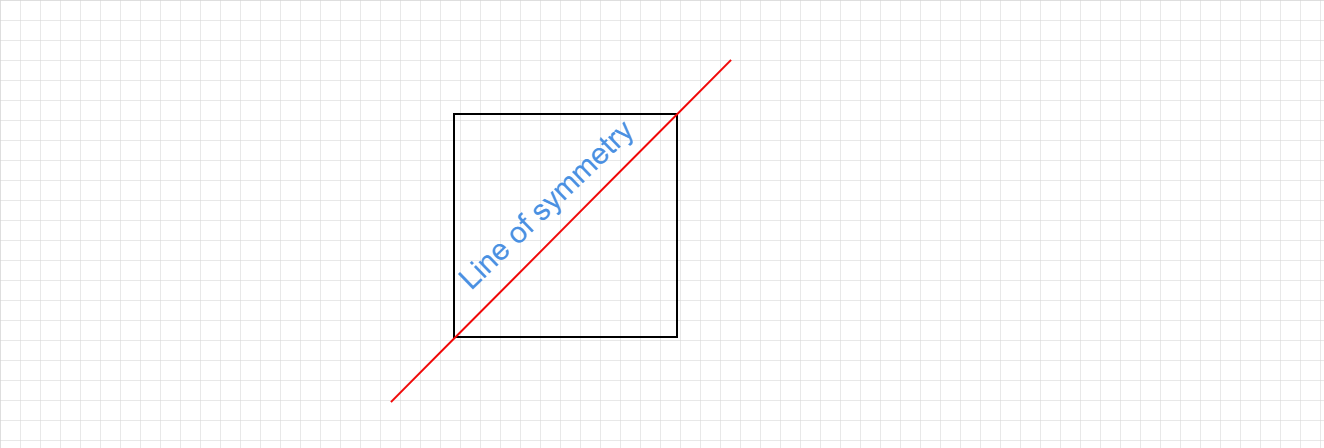# What is meant by Symmetry?

Symmetry :

• When an image looks identical to the original image after the shape is being turned or flipped, then it is called symmetry.
• Symmetry is a mirror image. It is a balanced and proportionate similarity found in two halves of an object, that is, one-half is the mirror image of the other half.

For example,

• When a square is cut along any one of its diagonals, the two triangles formed are symmetrical to each other.
• The imaginary line or axis along which you fold a figure to obtain the symmetrical halves is called the line of symmetry.
• It basically divides an object into two mirror-image halves. The line of symmetry can be vertical, horizontal, or diagonal.
• There may be one or more lines of symmetry. In the above example, the diagonal is the line of symmetry.Updated on: 10-Oct-2022

45 Views